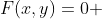1

## Great Free Online Math Tools

Published on Thursday, July 19, 2012 in , , , , , ,Exploring mathematical concepts is now easier and more impressive than ever before, thanks to computers and the internet.

In this post, we'll look at some incredible mathematical tools, all of which are available online for free!

Wolfram|Alpha - If you're not already familiar with Wolfram|Alpha, especially considering the numerous mentions I've made of it here on Grey Matters, you're in for a treat. Instead of simply searching for sites that may or may not answer your question, it simply tries to answer your question directly. I suggest watching Stephen Wolfram himself introduce the concept via video (Part 1, Part 2). You can get a better idea of its capabilities through the Wolfram|Alpha blog, examples, and tumblr blog, as well. You can even use your queries to develop embeddable widgets for your own website!

Google Calculator - Google's search engine has a built-in calculator. While it doesn't give you all the features of Wolfram|Alpha, it's still quite impressive. It can handle arithmetic, conversions, graphing, and more! Soople is a site with a graphical front end for Google calculator, so you can get a better idea of its capabilities.

Instacalc - No, this isn't as big a name as either of the first two, but that doesn't mean it's any less valuable. Instacalc is an online calculator designed to let you work with multi-step calculations. You can even embed your calculations, as I did in my post on Gas Math.

GeoGebra - GeoGebra is a VERY impressive CAS (Computer Algebra System). I include it here, as it runs primarily as a sort of Java web app, but is also available as an offline application. As seen in the video below, GeoGebra is primarily used to develop interactive “worksheets” that can help users explore mathematical concepts. For example, here's an interactive worksheet I developed to help Grey Matters readers explore the Estimating Square Roots technique I recently posted.

It's often also used to teach students mathematical concepts by having them develop interactive worksheets for themselves. If you're familiar with Wolfram's CDF format, as used in Wolfram|Alpha Pro and Mathematica, the concept is similar, except you can develop these for free, instead of paying hundreds of dollars. You develop your worksheet using graphics pages, an algebra view for managing variables, and even a spreadsheet.

The level of support for GeoGebra is awesome, especially considering it's a free program. There's a Wiki to help you learn how to use it, a site where you can download tens of thousands of worksheets (and upload your own!), a forum, and a wide variety of instructional videos to help you along.

CodeCogs Online LaTeX Equation Editor - LaTeX is a language used to define the typesetting features of documents, in such a way as to give consistent results across different platforms. It's found favor with mathematicians (Wolfram|Alpha's output is rendered with LaTeX), as it allows a more effective display of formulas and equations. For example, the following display is difficult to achieve in standard HTML, but easily achieved in LaTeX, with help from CodeCogs (click to see the LaTeX markup behind it):CodeCogs also offers a web interface for turning C or C++ code into online calculators (login required), and a LaTeX rendering engine for Excel.

Mathway - Mathway offers several online calculators, each geared to a specific mathematical subject, such as algebra, trigonometry, or calculus. It's entry method similar to CodeCog's LaTeX Equation Editor, but Mathway will work through the problem, as opposed to simply displaying it. With a free account, you can even save your calculations as worksheets to be used over and over again (free accounts see only the results, while paid accounts allow you to see the intermediate steps).

There are many more tools I can post, but the ones above are the current stand outs. If you program in Javascript, you might want to check out ASCIIsvg and/or JSXGraph.

Wikipedia provides an online calculator comparison chart here, and a computer algebra system comparison chart here, if you'd like to explore even more resources.

### 1 Response to Great Free Online Math Tools

Online tutoring is very helpful in solving the math problems and to understand the higher section topics.It can be very helpful for beginners because it provide practice and guidance for them.So that they will not face any kind of problem in future regarding further studies.